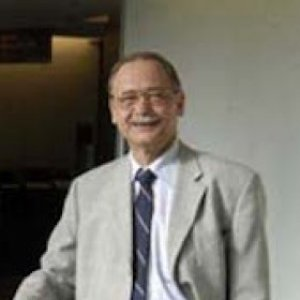# Ragnar-Olaf BuchweitzProfessor | University of Toronto, Department of Mathematics

Professor Buchweitz researches Commutative and Homological Algebra and Algebraic Geometry, Singularity Theory

2010

• English
• French
• German

## Articles (5)

#### Non-commutative desingularization of determinantal varieties, II: Arbitrary minors

International Mathematics Research Notices

2015 In our paper “Non-commutative desingularization of determinantal varieties I”, we constructed and studied non-commutative resolutions of determinantal varieties defined by maximal minors. At the end of the introduction, we asserted that the results could be ...

view more

#### On the derived category of Grassmannians in arbitrary characteristic

Compositio Mathematica

2015 In this paper we consider Grassmannians in arbitrary characteristic. Generalizing Kapranov's well-known characteristic-zero results, we construct dual exceptional collections on them (which are, however, not strong) as well as a tilting bundle. We show that this ...

view more

#### Strong global dimension of commutative rings and schemes

Journal of Algebra

2015 The strong global dimension of a ring is the supremum of the length of perfect complexes that are indecomposable in the derived category. In this note we characterize the noetherian commutative rings that have finite strong global dimension. We also give a ...

view more

#### The multiplicative structure on Hochschild cohomology of a complete intersection

Journal of Pure and Applied Algebra

2015 We determine the product structure on Hochschild cohomology of commutative algebras in low degrees, obtaining the answer in all degrees for complete intersection algebras. As applications, we consider cyclic extension algebras as well as Hochschild ...

view more

#### Hochschild (co-) homology of schemes with tilting object

Transactions of the American Mathematical Society

2013 Given a \$ k \$-scheme \$ X \$ that admits a tilting object \$ T \$, we prove that the Hochschild (co-) homology of \$ X \$ is isomorphic to that of \$ A=\ operatorname {End} _ {X}(T) \$. We treat more generally the relative case when \$ X \$ is flat over an affine scheme ...

view more